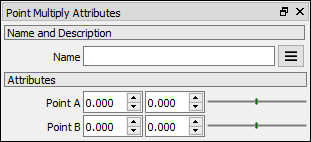# Point Multiply node

Left Toolbar >Math Nodes >Point Multiply

The Point Multiply node allows you to execute a multiplication operation on multiple Point values.

## Procedure

### To execute a multiplication operation on multiple Point Node Values in the node graph:

1. On the left toolbar choose Point Nodes> Point Multiply2. Drag the Point Multiply icon into the Node Graph.3. Connect the point Nodes to be multiplied to the input.

4. Connect the Output: result .

## Inputs

point A POINT type of value. This input grows automatically when a value is connected.

## Outputs

result Point Value (x,y).

## Attributes PanelPoint

Point A. This attribute is exposed as input by default. Right-click the Node to hide this attribute as input and set the point values manually.

Point B. This attribute is exposed as input by default. Right-click the Node to hide this attribute as input and set the point values manually.

Math Nodes

Main Page